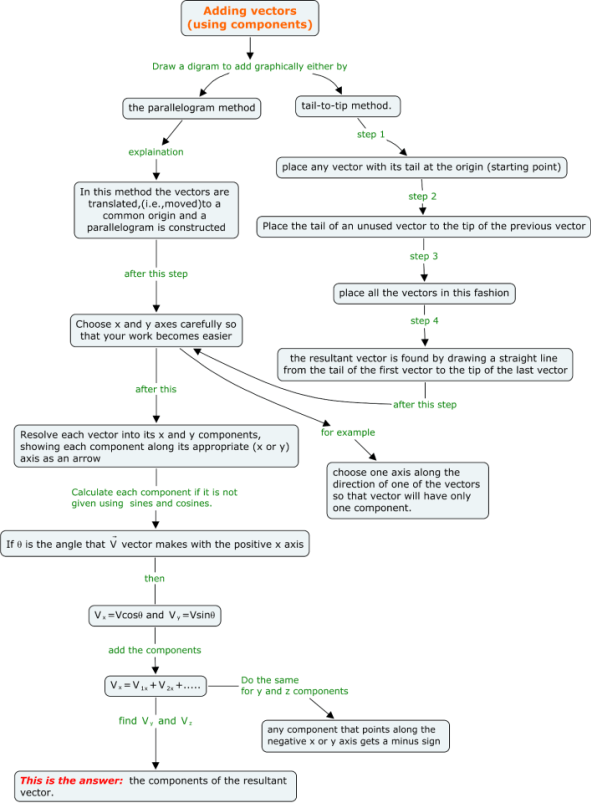# How to solve vector algebra problems

## How to solve vector algebra problems

This page is about how you can solve vector algebra problems easily. You will come across vectors in physics problem very frequently. So it is must to know to solve the vector mathematics in short time.And Making sure you have done in correctly.You will find vectors in every module be it mechanics, electrostatics, magentics
When you need to add or substract two or more vectors, you can use following procedure
• Select a coordinate system that is convenient. (Try to reduce the number of  components you need to find by choosing axes that line up with as many vectors as possible.)
• Draw a labeled sketch of the vectors described in the problem.
• Find the x and y components of all vectors
• Compute the resultants in x and y direction
• If necessary, use the Pythagorean theorem to find the magnitude of the resultant vector and select a suitable trigonometric function to find the angle that the resultant vector makes with the x axis.
I have made a concept map that shows how to do vector addition using components.

Concept map for vector addition using componentsProducts
• When u need to perform scalar or vector product.It is nice to following procedure
• Select a coordinate system(x,y,z) that is convenient. (Try to reduce the number of components you need to find by choosing axes that line up with as many vectors as possible.).
• Use i,j,k as the unit vector across x,y,z axis.
• Draw a labeled sketch of the vectors described in the problem.
• Express all the vector in form in i,j,k forms
• Perform the multiplication

## Related Topics

Go back to Class 12 Main Page using below links